# Light Reflection and Refraction class 10 Notes Science## myCBSEguide App

CBSE, NCERT, JEE Main, NEET-UG, NDA, Exam Papers, Question Bank, NCERT Solutions, Exemplars, Revision Notes, Free Videos, MCQ Tests & more.

CBSE class 10 Science Chapter 10 Light Reflection and Refraction notes in PDF are available for free download in myCBSEguide mobile app. The best app for CBSE students now provides Light Reflection and Refraction class 10 Notes latest chapter wise notes for quick preparation of CBSE board exams and school based annual examinations. Class 10 Science notes on Chapter 10 Light Reflection and Refraction are also available for download in CBSE Guide website.

## CBSE Guide Light Reflection and Refraction class 10 Notes

CBSE guide notes are the comprehensive notes which covers the latest syllabus of CBSE and NCERT. It includes all the topics given in NCERT class 10 Science text book. Users can download CBSE guide quick revision notes from myCBSEguide mobile app and my CBSE guide website.

## 10 Science notes Chapter 10 Light Reflection and Refraction

Download CBSE class 10th revision notes for Chapter 10 Light Reflection and Refraction in PDF format for free. Download revision notes for Light Reflection and Refraction class 10 Notes and score high in exams. These are the Light Reflection and Refraction class 10 Notes prepared by team of expert teachers. The revision notes help you revise the whole chapter in minutes. Revising notes in exam days is on of the best tips recommended by teachers during exam days.

CBSE Class 10 Science
Revision Notes
CHAPTER – 10
LIGHT REFLECTION & REFRACTION

Light is a form of energy, which gives us the power of vision.

In this chapter we will study the phenomena of reflection and refraction using the property of light i.e. straight line propagation (Light wave travel from one point to another, along a straight line).

Ray of light : It is a line in the direction of movement of light.
Beam of light : It is bucnh of rays of light.
Parallel beam : All the rays are parallel.

Reflection of Light
When the light is allowed to fall on highly polished surface, such as mirror, most of the light gets reflected.

Laws of Reflection

1. The angle of incidence is always equal to angle of reflection.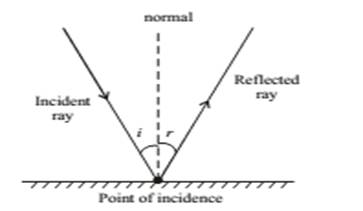2. The incident ray, reflected ray and the normal to the reflecting surface at the point of incidence lie in the same plane.

Image formed by Plane Mirror (Plane reflecting surface)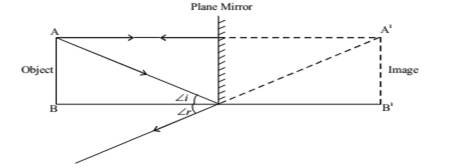1. Virtual (imaginary) & Erect : The image that do not form on screen.
Real images can be recorded on the screen.
2. Laterally inverted (The left side of object appear on right side of image)
3. The size of image is equal to that of object.
4. The image formed is as far behind the mirror as the object is in front of it.

Reflection of light by spherical Mirrors
Mirrors, whose reflecting surface are curved inward or outward spherically are called spherical mirror.
For example – Spoon  The curved surface of shinning spoon can be considered as curved mirror.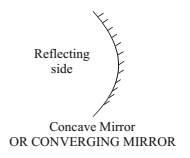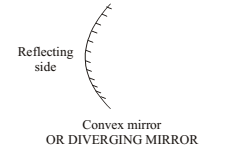If it is curved inward Act as concave mirror
If it is curved outward  Act as a convex mirror.

Few Basic terms related to Spherial Mirror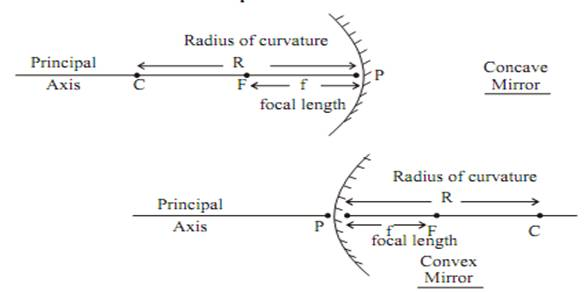1. Principal axis : Line joining the pole and centre of curvature of the spherical mirror.
2. Pole : The geometrical central point of the reflecting spherical surface. (aperture), denoted by (P).
3. Aperture : The width of reflecting spherical surface.
4. Centre of curvature : The centre of the hollow glass sphere of which the spherical mirror is a part is called as centre of curvature.
5. Radius of curvature : The distance between the pole and the centre of curvature. i.e.
PC = R or The radius of the hollow sphere of which the mirror is a part.
6. Focus point : The point on the principal axis, where all parallel rays meet after reflection is called as Principal Focus or Focus. It is denoted by letter ‘F’.
7. Focal length : The distance between the pole and focus point i.e. PF = f
8. Relationship between focal length and Radius of curvature.

Image Formation by Spherical Mirror

Before we learn the formation of image or ray diagram, let us go through few tips

(a) Remember, a ray of light which is parallel to principle axis always pass through focus (meet at focus) or vice-versa.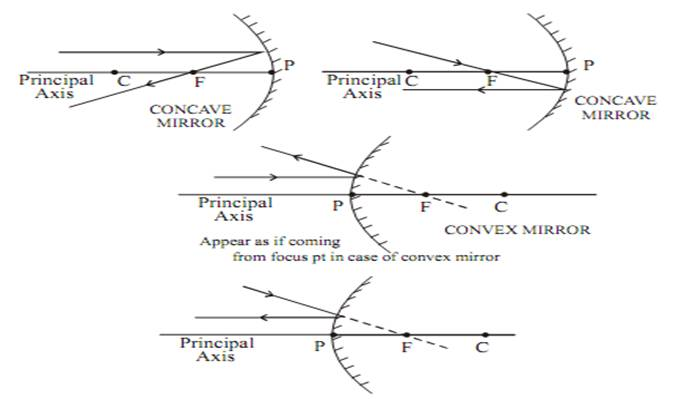(b) A ray of light which passes through centre of curvature (it is also known as normal at the point of incidence on spherical mirror) will retrace their path after reflection.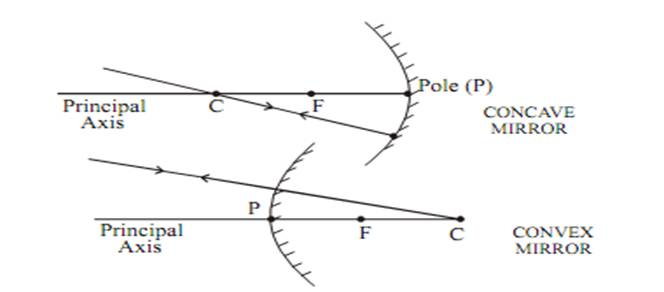(c) A ray of light falling on pole get reflected at the same angle on the other side of principal axis.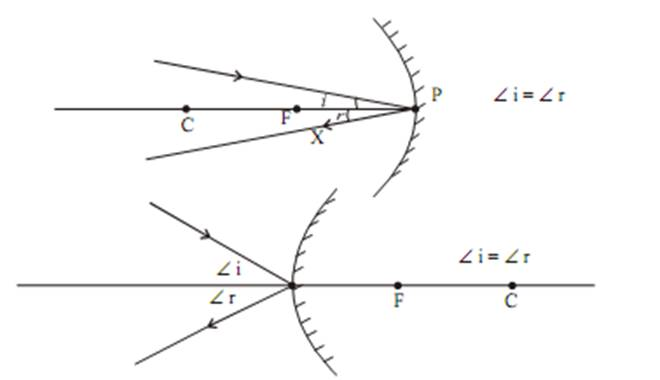Note : A ray of light passes through centre of curvature of reflecting spherical surface always act as normal at the point of incidence. If we know the normal we can draw angle of incidence and angle of reflection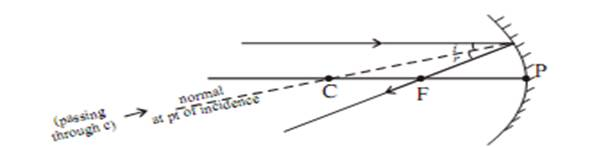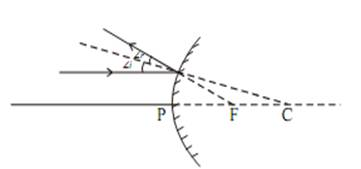Note 1 : The image will only form when two or more rays meets at a point. Image formation by a concave mirror for different position of the object.
The ray diagrams given in NCERT Books are also good to be followed.

Image Formation by Concave Mirror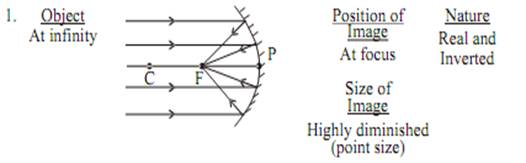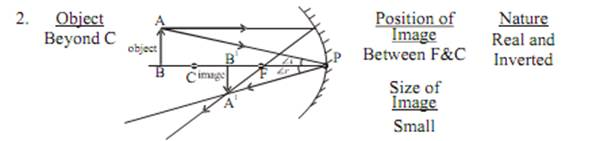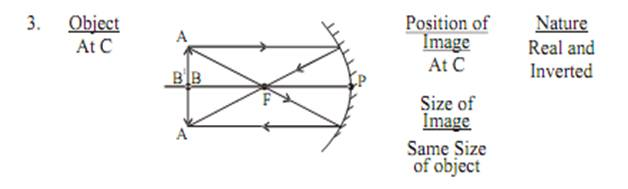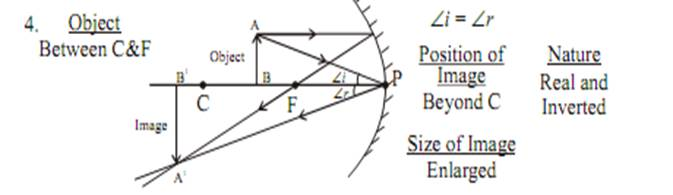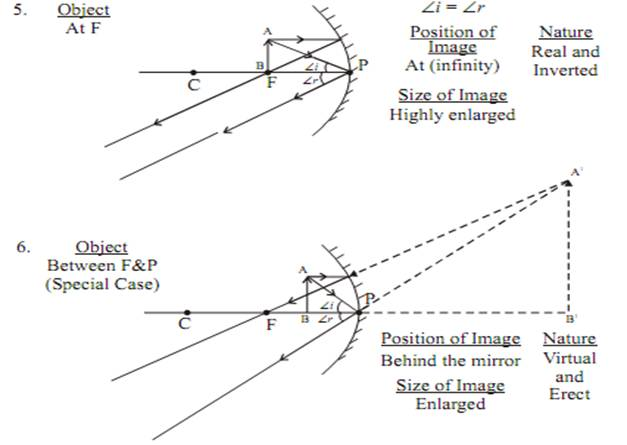Image formation by Convex Mirror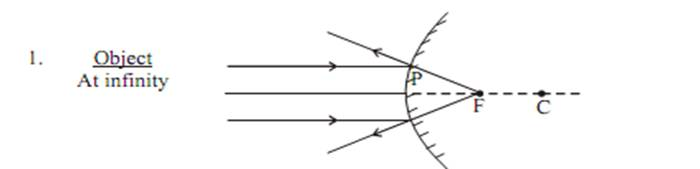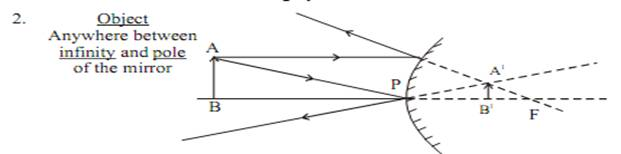Uses of Concave Mirror
1 Used in torches, search light and headlight of vehicle.
2. Used to see large image of face as shaving mirror
3. Used by dentist to see large images of the teeth
4. Large concave mirror used to focus sunlight (heat) in solar furnaces.

Uses of Convex Mirror
Used as rear-view mirror in vehicles because it gives erect image. Italso helps the driver to view large area.

Sign Convention for Reflection by Spherical Mirror

1. The object is always placed to the left side of mirror.
2. All distance should be measured from pole (P); parallel to principal axis.
3. Take ‘P’ as origin. Distances measured

Right of the origin (+x-Axis) are taken positive
Left of the origin (–x-Axis) are taken negative

Perpendicular to and above principal axis (+y-Axis) are taken positive
Perpendicular to and below principal axis (–y-Axis) are taken negative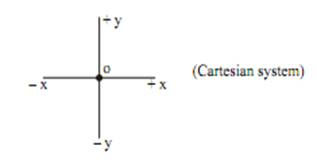Mirror Formula

distance between F and Pole
v  distance of image from Pole
distance of object from Pole
R  distance between centre of curvature and pole.

Where

Magnification

It is expressed as the ratio of the height of the image to height of the object.

From 1 and 2 equation

When  image height from principle axis.

Object height from principle axis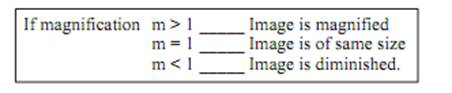Few tips to remember sign convention for Spherical mirror

 f u v CONCAVE -ve(real) -ve(real) -ve(real) +ve(virtual) CONVEX +ve +ve +ve

h – is always +ve
h´ – is +ve for virtual , –ve for Real.

Refraction of Light

Refraction of Light : Happens in Transparent medium when a light travels from one medium to another, refraction takes place.
A ray of light bends as it moves from one medium to another Refraction is due to change in the speed of light as it enters from one transparent medium to another.

Speed of light decreases as the beam of light travel from rarer medium to the denser medium.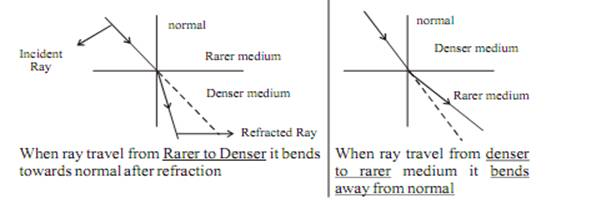Some Commonly observed phenomenon due to Refraction

• Rainbows.
• Light bending in a glass of water.
• Glasses.
• Camera lenses.
• Object dislocation in water.
• Binoculars..

Refraction through a Rectangular Glass Slab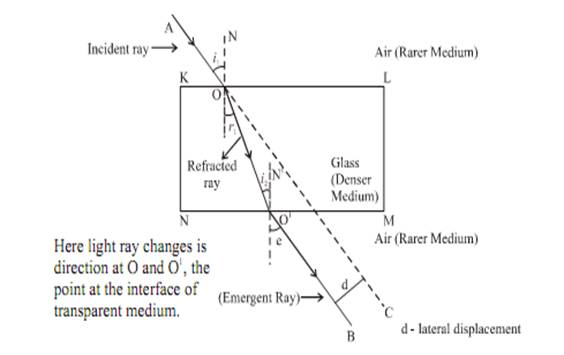When a incident ray of light AO passes from a rarer medium (air) to adenser medium (glass) at point. O on interface KL, it will bends towards the normal. At ptO1, on interface NM the light ray entered from denser medium(glass) to rarer medium (air) here the light ray will bend away from normal OO1 is a refracted ray OB is an emergent ray. If the incident ray is extended to C, we will observe that emergent ray O1B I parallel to incident ray. The ray will slightly displaced laterally after refraction.

Note : When a ray of light is incident normally to the interface of two media it will go straight, without any deviation.

Laws of Refraction of Light

The incident ray, the refracted ray and the normal to the interface of two transparent media at the point of incidence, all lie in the same plane.

• The ratio of sine of angle of incidence to the sine of angle of refraction is a constant i.e.

For given colour and pair of media, this law is also known as Snell’s Law

Constant n is the refractive index for a given pair of medium. It is the refractive index of the second medium with repect to first medium.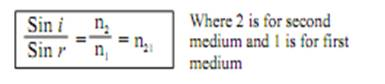Refractive Index

The refractive index of glass with respect to air is given by ratio of speed of light in air to the speed of light in glass.

c Speed of light in vacuum =  speed of light in air is marginally less, compared to that in vacuum.

Refractive index of air with respect to glass is given by

Refractive index of water

Refractive index of water

Spherical Lens

A transparent material bound by two surfaces, of which one or both surfaces are spherical, forms a lens.

Convex lens Concave lens
1. Bulging outwards 1. Bulging inwards.
2. Converging lens. 2. Diverging lens.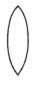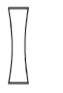Concave Lens
A lens bounded by two spherical surfaces, curved inwards is known asdouble concave lens (or simply concave lens)
It is also known as diverging lens because it diverges the light.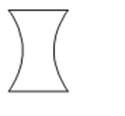Few Basic Terms Related to Spherical Lens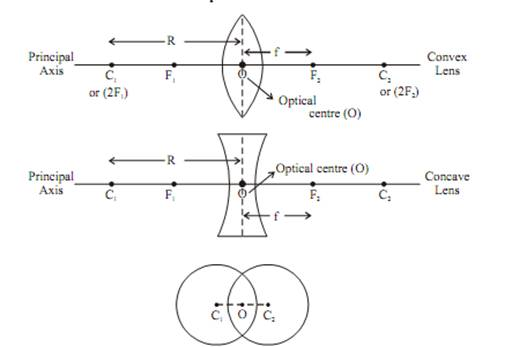1. Centre of curvature : A lens, either a convex lens or a concave lens is combination of two spherical surfaces. Each of these surfaces forma part of sphere. The centre of these two spheres are called centre of curvature represented by C1 and C2.
2. Principal axis :Imaginary straight line passing through the two centres of curvature
3. Optical Centre : The central point of lens is its optical centre (O). A ray of light, when passes through ‘O’ it remains undeviated i.e. it goes straight.
4. Aperture : The effective diameter of the circular outline of a spherical lens.
5. Focus of lens : Beam of light parallel to principal axis, after refraction from

1. Convex lens, converge to the point on principal axis, denoted by F, known as Principal focus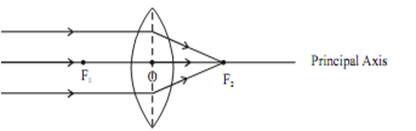2. Concave lens, appear to diverge from a point on the principal axis known as principal focus.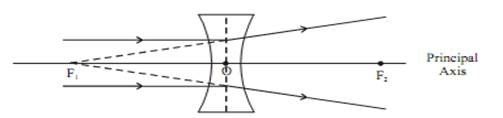The distance OF2 and OF1 is called as focal length

Tips for Drawaomg Ray Diagram

1. After refraction, a ray parallel to principal axis will pass through F.
2. A ray passes through F, after refraction will emerge parallel to principal axis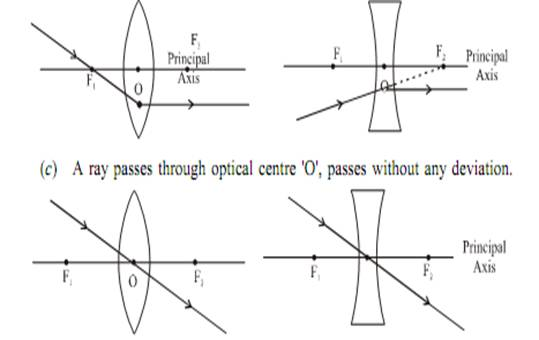Image formation by a convex lens for various position of object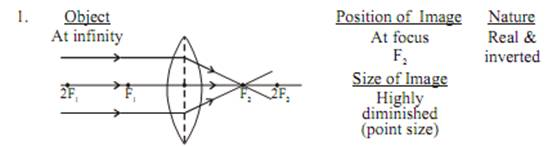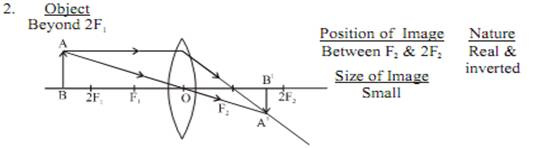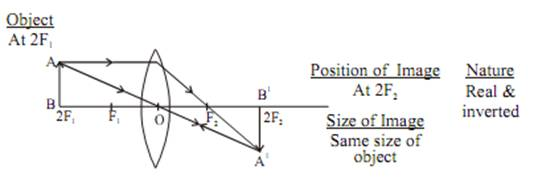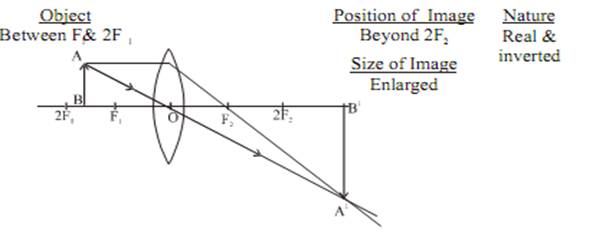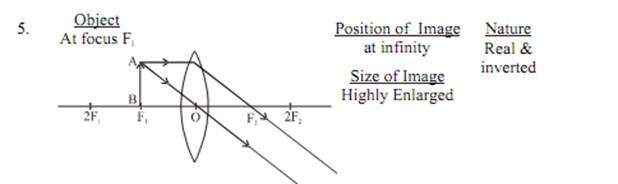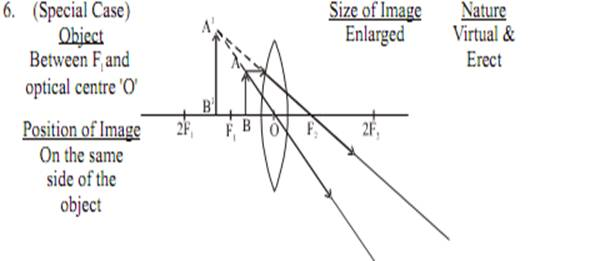Image Formation by Concave Lens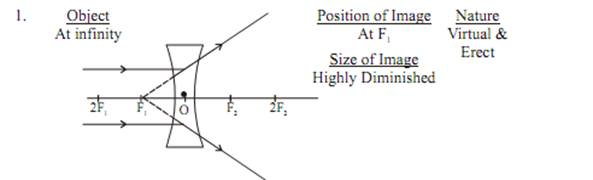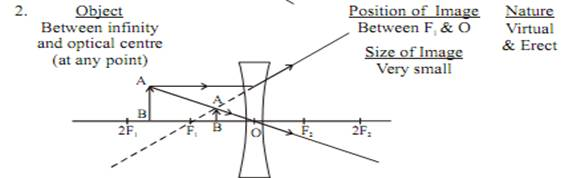Sign Convention for Refraction by Spherical Lens

Similar to that of spherical mirror, only the difference is that all themeasurement are made from optical centre ‘O’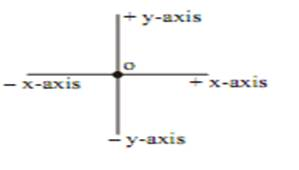Lens formula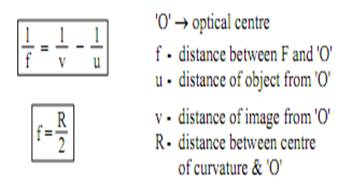Magnification

It is defined as the ratio of the height of image to the height of object.

It is also related to ‘u’ & ‘v’

From equation (1) & (2)
If magnification
m > 1,then image is magnified
m = 1 ,image is of same size
m < 1, image is diminished

Few Tips to Remember Sign Convention for Spherical Lens

 f u v CONCAVE -ve -ve -ve(virtual image always) CONVEX +ve -ve +ve(real) -ve(virtual)

h is always +ve
h´  –ve for Real and +ve for Virtual &Errect.

Power of Lens

The degree of convergence or divergence of light ray achieved by a lensis known as power of a lens.
It is defined as the reciprocal of its focal length Represented by P.
If F is given in meter, then

If F is given in cm , then

SI unit of power of a lens is “diopter” denoted by ‘D’
I diopter or ID  It is the power of lens whose focal length is I m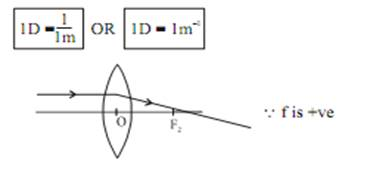Power of convave lens or diverging lens is always negative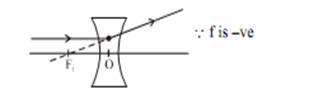If any optical instrument has many lens, then net power will be

## Light Reflection and Refraction class 10 Notes

• CBSE Revision notes for Class 10 Science PDF
• CBSE Revision notes Class 10 Science – CBSE
• CBSE Revisions notes and Key Points Class 10 Science
• Summary of the NCERT books all chapters in Science class 10
• Short notes for CBSE class 10th Science
• Key notes and chapter summary of Science class 10
• Quick revision notes for CBSE board exams

## CBSE Class-10 Revision Notes and Key Points

Light Reflection and Refraction class 10 Notes. CBSE quick revision note for Class-10 Science, Chemistry, Maths, Biology and other subject are very helpful to revise the whole syllabus during exam days. The revision notes covers all important formulas and concepts given in the chapter. Even if you wish to have an overview of a chapter, quick revision notes are here to do if for you. These notes will certainly save your time during stressful exam days.

To download Light Reflection and Refraction class 10 Notes, sample paper for class 10 Mathematics, Social Science, Science, English Communicative; do check myCBSEguide app or website. myCBSEguide provides sample papers with solution, test papers for chapter-wise practice, NCERT solutions, NCERT Exemplar solutions, quick revision notes for ready reference, CBSE guess papers and CBSE important question papers. Sample Paper all are made available through the best app for CBSE students and myCBSEguide website.### 4 thoughts on “Light Reflection and Refraction class 10 Notes Science”

1. perfect concept

2. very helpful tq for the notes

3. Nice app helpful at many times to it is recommended to all students

4. thanks a lot . very helpful